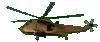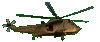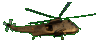Complete a Square     Factoring Help      Fun Game Tips:

- A perfect square is formed by multiplying a quantity times itself, as in (3)(3) =9 .
Here are seven perfect squares: 25,  144,  169,  (-3)2,  r2,  (y+3)2,   (2w-5t)2 .
- The 'Perfect Trinomial Square'  x2+6x+9  can be factored as (x+3)(x+3).

- When given only 2 terms such as  x2+6x  , how can the 3rd term '9' be found to complete the square?
The answer is to take half of the '6' to get '3' and square the '3' to get the '9'.
When you complete the square for  x2+6x  you use  x2+6x+(6/2)2  for the answer  x2+6x+9  .
Sample: if x=10, show 160=(10)2+6(10) changes to the square 169=(10)2+6(10)+(6/2)2 by adding '9'.

- In general, to complete the square for   x2+bx   , take half of the b and square it
to get the 3rd term as shown here   x2+bx+(b/2)2  .
To complete  x2+5x  , the answer  x2+5x+(2.5)2  is the trinomial square  x2+5x+6.25  .

- When the numerical coefficient of x2 is not a simple 'one', for example  2x2+12x  , in order
to complete the square for  2x2+12x  , begin with the common factor '2' to get   2[x2+6x]
and then take half of the 6 and square it, to get the trinomial square   2[x2+6x+(6/2)2]  =  2[x2+6x+9] .

- In general, to complete the square for  ax2+bx  , use common factoring as in  a[x2+(b/a)x] , then
take half of b/a and square it, (b/2a)2, to get the perfect trinomial square  a[x2+(b/a)x+(b/2a)2] .

- Squared quantities are used in formulas for many applications: light and radiation intensity,
gravitational attraction, kinetic energy, bending strength, maximum & minimum problems...

- Completing the square can be used to find the lowest point [vertex] of a parabola such as y = x2+6x+8.
For example, complete the square for y = x2+6x+8 based on squaring half of the '6'.
y = x2+6x+9-9+8.    Add '9' and also subtract '9' to keep the y value unchanged.
y = (x+3)2-9+8.
y = (x+3)2-1.
Since the perfect square (x+3)2 is always positive, then the lowest value of 'y' is '-1'.
The vertex of this sample parabola is (-3,-1).  The same parabola can be represented as y+1 = (x+3)2.